Test: Electromagnetics - 1

# Test: Electromagnetics - 1

Test Description

## 15 Questions MCQ Test GATE ECE (Electronics) 2023 Mock Test Series | Test: Electromagnetics - 1

Test: Electromagnetics - 1 for Electronics and Communication Engineering (ECE) 2023 is part of GATE ECE (Electronics) 2023 Mock Test Series preparation. The Test: Electromagnetics - 1 questions and answers have been prepared according to the Electronics and Communication Engineering (ECE) exam syllabus.The Test: Electromagnetics - 1 MCQs are made for Electronics and Communication Engineering (ECE) 2023 Exam. Find important definitions, questions, notes, meanings, examples, exercises, MCQs and online tests for Test: Electromagnetics - 1 below.
Solutions of Test: Electromagnetics - 1 questions in English are available as part of our GATE ECE (Electronics) 2023 Mock Test Series for Electronics and Communication Engineering (ECE) & Test: Electromagnetics - 1 solutions in Hindi for GATE ECE (Electronics) 2023 Mock Test Series course. Download more important topics, notes, lectures and mock test series for Electronics and Communication Engineering (ECE) Exam by signing up for free. Attempt Test: Electromagnetics - 1 | 15 questions in 45 minutes | Mock test for Electronics and Communication Engineering (ECE) preparation | Free important questions MCQ to study GATE ECE (Electronics) 2023 Mock Test Series for Electronics and Communication Engineering (ECE) Exam | Download free PDF with solutions
 1 Crore+ students have signed up on EduRev. Have you?
Test: Electromagnetics - 1 - Question 1

### Assertion (A) : Smith chart is designed for λ/2length only. Reason (R) : For every λ/2 length, the load impedance appears to be same as Zin.

Test: Electromagnetics - 1 - Question 2

### Stub matching is used to match the load to characteristic impedance. Stub should be placed such that the admittance value at that point is

Detailed Solution for Test: Electromagnetics - 1 - Question 2

Stub matching is an effective technique in order to cancel out the reflectors when placed such that its reactive admittance value at the location of the stub is '0'. [Then it minimize losses]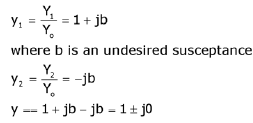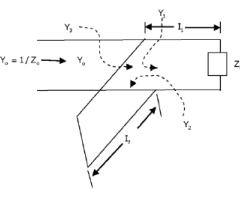Test: Electromagnetics - 1 - Question 3

### Match the following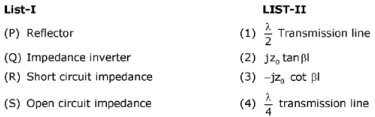Detailed Solution for Test: Electromagnetics - 1 - Question 3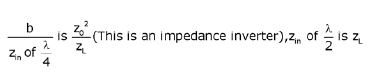Test: Electromagnetics - 1 - Question 4

The characteristic impedance of a lossless transmission line at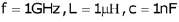is _________________ (ohms)

Detailed Solution for Test: Electromagnetics - 1 - Question 4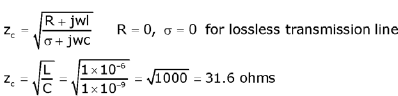Test: Electromagnetics - 1 - Question 5

Assertion (A)    :    Magnetic field is continuous and always rotational.
Reason (R)    :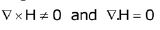Detailed Solution for Test: Electromagnetics - 1 - Question 5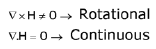So assertion and reason both are correct and reason is the correct explanation for assertion.

Test: Electromagnetics - 1 - Question 6

An electric wave propagating through a lossless medium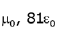is given by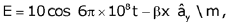:the phase constant β is given by

Detailed Solution for Test: Electromagnetics - 1 - Question 6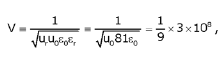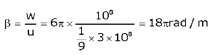Test: Electromagnetics - 1 - Question 7

Assertion (A)    :    Polarization is always defined in terms of electric field orientation.
Reason (R)    :    Magnetic field is due to induced effect of electric field so we cannot measure it directly.

Detailed Solution for Test: Electromagnetics - 1 - Question 7

Assertion and reason are correct and reason is the correct explanation for assertion

Test: Electromagnetics - 1 - Question 8

Consider a wave which is having a phase velocity of 3x108m/s .Find the group ✓elocitv and the medium in which it is travelling

Detailed Solution for Test: Electromagnetics - 1 - Question 8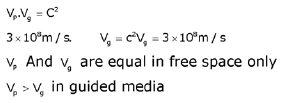Test: Electromagnetics - 1 - Question 9

Phase difference between E and H in a high loss medium is _______________ (Degrees)

Detailed Solution for Test: Electromagnetics - 1 - Question 9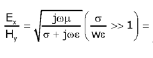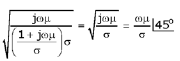Test: Electromagnetics - 1 - Question 10

On the smith chart the location of the normalized load point is at 0.15λ, with reference to wavelength towards generator (WTG), scale operating wavelength is 4 cm. The location of first vmax from load is

Detailed Solution for Test: Electromagnetics - 1 - Question 10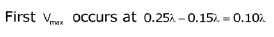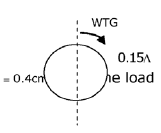Test: Electromagnetics - 1 - Question 11

Which of the following statements on a smith chart are correct?
1.    Moving in clockwise direction is analogous to moving towards generator.
2.    VSWR circles can be plotted on a smith chart.
3.    Smith chart is the superposition of locus of VSWR and reflection coefficients.
4.    To complete a distance of 0.75λ. it's enough to move 0.5λ. towards aenerator.

Detailed Solution for Test: Electromagnetics - 1 - Question 11

Smith chart is superposition of locus of resistance and reactance circles.

Test: Electromagnetics - 1 - Question 12

S-matrix for two port transmission line is given by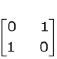. The reflection
coefficient and the transmission power through port 1 for input power of 5 mW is

Detailed Solution for Test: Electromagnetics - 1 - Question 12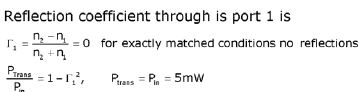Test: Electromagnetics - 1 - Question 13

Match the following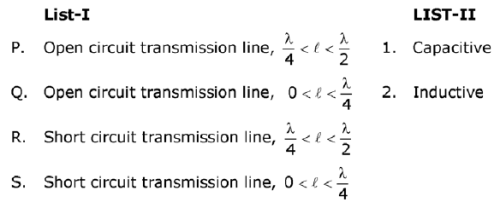Test: Electromagnetics - 1 - Question 14

For a waveguide component whose VSWR is 1.4 when the component is terminated with a matched load. The power to the matched load is 25 mW for an input power of 100 mW. The same results are obtained when component is reversed, then the S-matrix is

Detailed Solution for Test: Electromagnetics - 1 - Question 14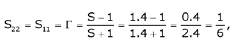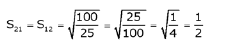Test: Electromagnetics - 1 - Question 15

The propagation constant of a transmission line with impedance and admittance of 9 and 16 respectively is

Detailed Solution for Test: Electromagnetics - 1 - Question 15

The propagation constant is given by γ = √(ZY), where Z is given by 9 and Y is 16. On substituting the given values, the propagation constant will be γ = √(ZY) = √(9 x 16) = 12 units.

## GATE ECE (Electronics) 2023 Mock Test Series

21 docs|263 tests
Information about Test: Electromagnetics - 1 Page
In this test you can find the Exam questions for Test: Electromagnetics - 1 solved & explained in the simplest way possible. Besides giving Questions and answers for Test: Electromagnetics - 1, EduRev gives you an ample number of Online tests for practice

## GATE ECE (Electronics) 2023 Mock Test Series

21 docs|263 tests(Scan QR code)